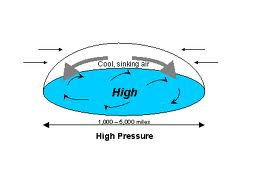# to model "pressure force" per unit volume (Navier)

The "pressure" (especially the continous pressure in the context of airfoil uplift theory in an ideal fluid, which is vortex-free per definition) is a mathematical arte-fact, while the "gradient of pressure" is a force per unit volume. We propose to represent the "pressure force" by the components of a corresponding Plemelj-Stieltjes potential, which requires less regularity assumptions than current standard model.

We claim, that the route cause of still unsolved open questions of the NSE is not related to the specifics of non-linear and/or the non-stationary obstacles, it is due to the fact, that by design it can not be assigned to one of the elliptic, parabolic or hyperbolic PDE clusters. This is due to the fact, that the model puts velocity and pressure into a certain relationship, which leads to mathematical incompatibilities. Those become visible, when it's possible to decouple the NSE equations into separate PDEs for velocity and pressure.The proposed model for a Mie theory accompanied by the concpet of an electric pressure overcomes this issue.

From G. Galdi we quote:

"the field p(x,t) can be formally obtained - by operating with "div" on both sides of the NSE - as a solution of a Neumann problem. From this it is clear that to describe the values of the pressure at the bounding walls or at the Initial time independently of v, could incompatible with the NSE and, therefore, could render the problem ill-posed".

The Gauss integral theorem states, that the integral of the normal derivative of a harmonic function vanishes. This is intuitively obvious if one interprets u as a velocity potential. Then the Gauss integral theorem means that for incompressible, irrotational flow with no source, the net mass flow through a prescribed boundary is zero.

Proposition

The solution of boundary integral operator equations (especially the hyper singular operator in the context of the Laplace equation (S. Amini), which is the normal derivative of the double layer potential) is proposed to be applied alternatively to define a non-harmonic pressure function p. With respect to the Gauss theorem this means, that the pre-requisite of an irrotational flow is omitted.

In other words, it is basically about an alternative representation/ definition of the pressure function, not as a solution of the Neumann problem with corresponding (too high) regularity requirements, but as solution of a boundary integral operator equation, which "allows" the "rotation" of fluids.

With respect to the defintion of the (singular) integral operator we refer to Kevorkian J., Partial Differential Equations, (2.12.2), p. 98)

With respect to the Fourier transform of the uniform distribution of unit mass over the untit sphere with centre at the origin for n=2, which is the Bessel function of first kind J(0)(x) we refer to

B. E. Petersen, "Introduction to the Fourier Transform and Pseudo-Differential Operators", example 2.5.Kevorkian J., Partial Differential Equations, Analytical Solution Techniques